# Generalized Linear Model III¶

Dec. 4, 2018 $\newcommand{\bs}{\boldsymbol}$ $\newcommand{\argmin}{\underset{\bs{#1}}{\text{arg min}}\,}$ $\newcommand{\argmax}{\underset{\bs{#1}}{\text{arg max}}\,}$ $\newcommand{\tr}{^{\top}}$ $\newcommand{\norm}{\left|\left|\,#1\,\right|\right|}$ $\newcommand{\given}{\,|\,}$ $\DeclareMathOperator{\E}{\mathbb{E}}$ $\newcommand{\blue}{{\color{blue} {#1}}}$ $\newcommand{\red}{{\color{red} {#1}}}$ $\newcommand{\orange}{{\color{orange} {#1}}}$ $\newcommand{\pfrac}{\frac{\partial #1}{\partial #2}}$

We are now ready to improve the spatial model proposed in the previous post. Recall that for the $j$-th group, where $j=1,...,m$, we assumed that

$$y_j^{(i)} = \bs{\theta}\tr \bs{x}_j^{(i)} + \bs{\gamma}_j\tr \bs{z}_j^{(i)} + \epsilon_j^{(i)}, \tag{1}$$

where $\bs{\theta}$ is the fixed effect and $\bs{\gamma}_j$ are the random effect.

We proposed the naive model,

$$\bs{Y} = \bs{X}\bs{\theta} + \bs{Z}\bs{b} + \bs{\epsilon}.$$

where $\bs{Z}$ is the location matrix whose rows are basis vectors encoded for each location $j$. Componentwise, this model is equivalent to

$$y_j^{(i)} = \bs{\theta}\tr \bs{x}_j^{(i)} + b_j + \epsilon_j^{(i)}, \tag{2}$$

for $i = 1,..., n_j$, where $n_j$ is the number of training examples in group $j$. The term $b_j$ is the $j$-th component of the vector $\bs{b}$, which is assumed to follow the Conditional Autoregressive Model (CAR), i.e.,

\begin{aligned} \bs{b} &\sim \text{CAR}(\sigma^2\tau^2, \rho) \\ &\sim \mathcal{N}(\bs{0},\, \sigma^2\tau^2(\bs{D}-\rho\bs{W})^{-1}) \end{aligned}\tag{3}

where $\rho$ controls the strength of spatial dependence, and $\bs{W} = (W_{i,j})$ is the neighborhood matrix, such that

$$W_{i,j} = \begin{cases} 1 & \text{ if } i \text{ neighbors } j \\ 0 & \text{ otherwise. } \end{cases}$$

And $\bs{D} = \text{diag}(D_{i,i})$ is a diagonal matrix such that

$$D_{i,i} = \sum_j W_{i,j}.$$

However, the model (2) is limited since the random effect is only included in the intercept term, and is independent from the covariates. We are ready to generalize the ICAR model.

## The Augmented CAR Model¶

Let us write out the parameters:

\begin{aligned} \bs{\theta} &= (\theta_0, \theta_1, ..., \theta_p)' \\ \bs{x}_j^{(i)} &= (1, x_{j1}^{(i)}, ..., x_{jp}^{(i)})' \end{aligned}

So our model (2) becomes

$$y_j^{(i)} = \theta_0 + b_j + \theta_1 x_{j1}^{(i)} + \cdots + \theta_p x_{jp}^{(i)} + \epsilon_j^{(i)}.$$

Inspired by this form, we propose the following addition:

$$y_j^{(i)} = (\theta_0+ b_{j0}) + (\theta_1 + b_{j1}) x_{j1}^{(1)} + \cdots + (\theta_p + b_{jp}) x_{jp}^{(i)} + \epsilon_j^{(i)}, \tag{4}$$

where

\begin{aligned} \bs{b}_0 &= (b_{10},..., b_{m0})' \\ \bs{b}_1 &= (b_{12},..., b_{m2})' \\ & \vdots \\ \bs{b}_{p} &= (b_{1p},..., b_{mp})' \end{aligned}

such that

$$\bs{b}_0, ..., \bs{b}_{p} \overset{i.i.d.}{\sim} \text{CAR}(\sigma^2 \tau^2, \rho). \tag{5}$$

To express (4) in a vectorized notation, define

$$\bs{Y}_j = (y_j^{(1)},..., y_j^{(n_j)})'. \tag{6}$$

$$\bs{X}_j = \begin{bmatrix} & (\bs{x}_j^{(1)})\tr & \\ & \vdots & \\ & (\bs{x}_j^{(n_j)})\tr & \end{bmatrix}, \quad \bs{\beta}_j = \begin{bmatrix} b_{j0} \\ \vdots \\ b_{jp} \end{bmatrix}. \tag{7}$$

Combining (4), (5), and (6), we have

$$\bs{Y}_j = \bs{X}_j(\bs{\theta} + \bs{\beta}_j) + \bs{\epsilon}_j. \tag{8}$$

The model (7) can be expressed in the augmented form by defining:

$$\bs{Y} = \begin{bmatrix} \bs{Y}_1 \\ \vdots \\ \bs{Y}_m \end{bmatrix}, \quad \bs{X}_{(a)} = \begin{bmatrix} \bs{X}_1 & & & \\ & \bs{X}_2 & & \\ & & \ddots & \\ & & & \bs{X}_m \end{bmatrix}, \quad \bs{\beta}_{(a)} = \begin{bmatrix} \bs{\theta}+\bs{\beta}_1 \\ \bs{\theta}+\bs{\beta}_2 \\ \vdots \\ \bs{\theta}+\bs{\beta}_m \end{bmatrix}, \tag{9}$$

where the subscript $(a)$ denotes augmented.

Finally, the model can be written as

$$\bs{Y} = \bs{X}_{(a)} \bs{\beta}_{(a)} + \bs{\epsilon}. \tag{10}$$

Suppose we have

$$\bs{\theta} \sim \mathcal{N}(\bs{0}, \bs{R}), \tag{11}$$

Hence, the prior distribution of the augmented parameter vector $\bs{\beta^{(a)}}$ is

\begin{aligned} \bs{\beta}_{(a)} &\sim \mathcal{N}\left(\bs{0}, \bs{\Sigma} \right) \\ \text{where}\,\, \bs{\Sigma} &= \bs{P}(\text{diag}_m(\bs{R}) + \text{diag}_{p+1}(\sigma^2\tau^2(\bs{D}-\rho\bs{W})^{-1})\bs{P}\tr. \end{aligned}\tag{12}

where $\text{diag}_m (\bs{R})$ denotes a diagonal matrix consisting of $m$ equal blocks $\bs{R}$, and $\bs{P}$ is the permutation matrix such as

$$\begin{bmatrix}\bs{\beta}_1 \\ \vdots \\ \bs{\beta}_m \end{bmatrix} = \bs{P}\begin{bmatrix} \bs{b}_0 \\ \vdots \\ \bs{b}_p \end{bmatrix}. \tag{13}$$

For instance, if $p=m=2$, then

$$\bs{\beta}_1 = \begin{bmatrix} b_{10} \\ b_{11} \\ b_{12} \end{bmatrix}, \quad \bs{\beta}_2 = \begin{bmatrix} b_{20} \\ b_{21} \\ b_{22} \end{bmatrix},$$

and the permutation matrix is

$$\bs{P} = \begin{bmatrix} \red{1} & 0 & 0 & 0 & 0 & 0 \\ 0 & 0 & \red{1} & 0 & 0 & 0 \\ 0 & 0 & 0 & 0 & \red{1} & 0 \\ 0 & \red{1} & 0 & 0 & 0 & 0 \\ 0 & 0 & 0 & \red{1} & 0 & 0 \\ 0 & 0 & 0 & 0 & 0 & \red{1} \end{bmatrix}.$$

The model is complete by specifying following distributions.

\begin{aligned} \bs{\epsilon} &\sim \mathcal{N}(\bs{0}, \sigma^{2} \bs{I}) \\ \sigma^{-2} &\sim \text{Gamma}(a_{\sigma}, b_{\sigma}) \\ \tau^{-2} &\sim \text{Gamma}(a_{\tau}, b_{\tau}). \end{aligned}\tag{14}

Now, we can write down the joint posterior distribution as follows.

\begin{aligned} \pi(\bs{\beta}_{(a)}, \sigma^{-2}, \tau^{-2}, \rho) &\propto (\sigma^{-2})^{\frac{n}{2}} \text{exp}\left(\frac{-(\bs{Y}-\bs{X}_{(a)}\bs{\beta}_{(a)})\tr(\bs{Y}-\bs{X}_{(a)}\bs{\beta}_{(a)})}{2\sigma^{2}}\right) \\ & \quad \times \text{exp}\left(\frac{-\bs{\beta}_{(a)}\tr\bs{\Sigma}^{-1}\bs{\beta}_{(a)}}{2}\right)\\ & \quad \times (\sigma^{-2})^{a_{\sigma}-1} \text{exp}(-\sigma^{-2} b_{\sigma}) \\ & \quad \times (\tau^{-2})^{a_{\tau}-1} \text{exp}(-\tau^{-2} b_{\tau}) \\ \text{where}\,\, \bs{\Sigma} &= \bs{P}(\text{diag}_{p+1}(\sigma^2\tau^2(\bs{D}-\rho\bs{W})^{-1})\bs{P}\tr. \end{aligned} \tag{15}

where we have removed the prior parameter $\bs{R}$ to simplify computation, as the prior effect can be "absorbed" into the spatial random effect.

## The Model¶

Let's start by finding the full conditional distribution of the augmented coefficient $\bs{\beta}^{(a)}$.

\begin{aligned} \pi(\bs{\beta}_{(a)}) &\propto \text{exp}\left(-\frac{1}{2\sigma^{2}}\left((\bs{Y} - \bs{X}_{(a)}\bs{\beta}_{(a)})\tr(\bs{Y}-\bs{X}_{(a)}\bs{\beta}_{(a)}) + \sigma^{2}\bs{\beta}_{(a)}\tr \bs{\Sigma}^{-1}\bs{\beta}_{(a)}\right)\right)\\ &\propto \text{exp}\left(-\frac{1}{2\sigma^{2}}\left(\bs{\beta}_{(a)}\tr(\bs{X}_{(a)}\tr\bs{X}^{(a)} + \sigma^{2}\bs{\Sigma}^{-1})\bs{\beta}_{(a)}) - 2{\bs{Y}}\tr \bs{X}_{(a)}\bs{\beta}_{(a)}\right)\right) \end{aligned} \tag{16}

We compare this kernel to the kernel of a multivariate normal $\bs{Z} \sim \mathcal{N}(\bs{\mu}, \sigma^{2}\bs{\Sigma})$ which has the form

\begin{aligned} f_{\bs{Z}} &\propto \text{exp}\left(-\frac{1}{2\sigma^{2}}(\bs{Z}-\bs{\mu})\tr \bs{\Sigma}^{-1}(\bs{Z}-\bs{\mu})\right) \\ &= \text{exp}\left(-\frac{1}{2\sigma^{2}}(\bs{Z}\tr \bs{\Sigma}^{-1}\bs{Z} - 2\bs{Z}\tr \bs{\Sigma}^{-1}\bs{\mu} + \bs{\mu}\tr\bs{\Sigma}^{-1}\bs{\mu})\right) \\ & \propto \text{exp}\left(-\frac{1}{2\sigma^{2}}(\bs{Z}\tr\bs{\Sigma}^{-1}\bs{Z}-2\bs{\mu}\tr\bs{\Sigma}^{-1}\bs{Z}) \right). \end{aligned} \tag{17}

Comparing (16) with (17), we conclude that

$$\bs{\beta}_{(a)} \sim \mathcal{N}\left((\bs{X}_{(a)}\tr\bs{X}_{(a)} + \sigma^{2}\bs{\Sigma}^{-1})^{-1} \bs{X}_{(a)}\tr\bs{Y}, \quad \sigma^{2}(\bs{X}_{(a)}\tr\bs{X}_{(a)}+\sigma^{2}\bs{\Sigma}^{-1})^{-1}\right). \tag{18}$$

Now in terms of deriving full conditionals for $\sigma^{-2}$ and $\tau^{-2}$ and $\rho$, we are out of luck! Instead, we have to rely on the Metropolis-Hastings algorithm. To do that, let's write down the log-likelihoods:

\begin{aligned} l(\sigma^{-2}) &\propto -\frac{1}{2}\bs{\beta}_{(a)}\tr\bs{\Sigma}^{-1}\bs{\beta}_{(a)} + (a_{\sigma}-1)\log(\sigma^{-2}) - \sigma^{-2} b_{\sigma} \\ l(\tau^{-2}) &\propto -\frac{1}{2}\bs{\beta}_{(a)}\tr\bs{\Sigma}^{-1}\bs{\beta}_{(a)} + (a_{\tau}-1)\log(\tau^{-2}) - \tau^{-2} b_{\tau} \end{aligned} \tag{19}

Next, we need to find proposal distributions. Since all parameters of interest are nonnegative, we can naively choose the following proposal distributions:

\begin{aligned} J(\sigma^{-2} \given \sigma^{-2,(i)}) &\sim \text{Gamma}(\alpha_1, \sigma^{-2,(i)}/\alpha_1) \\ J(\tau^{-2} \given \sigma^{-2,(i)}) &\sim \text{Gamma}(\alpha_2, \tau^{-2,(i)}/\alpha_2) \end{aligned} \tag{20}

where $\alpha_1, \alpha_2$ are turning parameters, and $\sigma^{-2,(i)}, \tau^{-2,(i)}$ are the parameter values in the previous iteration of the Metropolis Hastings sampling. We can now build functions that outputs acceptance probabilities below.

In :
library(Matrix) # for diagnal sparse matrix
library(dplyr)

# Parameters
# -----------
# P = permutation matrix
# sig2, tau2 = sigma^-2, tau^-2
# D, rho, W = CAR model parameters
# as, bs = prior parameters for sig2
# at, bt = prior parameters for tau2
# beta = augmented regression coefficient

# computes inverse of Sigma
Sig.inv <- function(P, sig2, tau2, D, rho, W){
p <- dim(R) # size of beta
m <- dim(W) # number of spatial units
M <- bdiag(replicate(p, (D-rho*W)*sig2*tau2, simplify=FALSE))
solve(t(P))%*%M%*%solve(P)
}

In :
# log-likelihoods of sig2
sig2.log <- function(sig2, P, tau2, D, rho, W, beta, as, bs) {
sig.inv <- Sig.inv(P, sig2, tau2, D, rho, W)
(-t(beta)%*%sig.inv%*%beta/2 + (as-1)*log(sig2) - bs*sig2)
}

# log-likelihoods of tau2
tau2.log <- function(tau2, P, sig2, D, rho, W, beta, at, bt) {
sig.inv <- Sig.inv(P, R, sig2, tau2, D, rho, W)
(-t(beta)%*%sig.inv%*%beta/2 + (at-1)*log(tau2) - bt*tau2)
}

In :
# acceptance probability for sig2
alpha.sig2 <- function(sig2.new, sig2.old, P, tau2, D, rho, W, beta, as, bs, alpha2) {
top <- {sig2.log(sig2.new, P, tau2, D, rho, W, beta, as, bs) +
dgamma(sig2.old, alpha2, sig2.new/alpha2, log=TRUE)}
bot <- {sig2.log(sig2.old, P, tau2, D, rho, W, beta, as, bs) +
dgamma(sig2.new, alpha2, sig2.old/alpha2, log=TRUE)}
alpha <- min(1, exp(top-bot))
rbinom(1,1,alpha)
}

# acceptance probability for tau2
alpha.tau2 <- function(tau2.new, tau2.old, P, sig2, D, rho, W, beta, at, bt, alpha3) {
top <- {tau2.log(tau2.new, P, sig2, D, rho, W, beta, at, bt) +
dgamma(tau2.old, alpha3, tau2.new/alpha3, log=TRUE)}
bot <- {tau2.log(tau2.old, P, sig2, D, rho, W, beta, at, bt) +
dgamma(tau2.new, alpha3, tau2.old/alpha3, log=TRUE)}
alpha <- min(1, exp(top-bot))
rbinom(1,1,alpha)
}


Recall from my previous post, I talked about a quick method for sampling from multivariate normal distribution $\mathcal{N}(\bs{A}^{-1}\bs{a}, \bs{A}^{-1})$. We can use it here.

In :
# Efficient sampling from multivariate normal
sample.normal <- function(a, A){
#   sampling from N(A^-1*a, A^-1)
n <- length(a)
Lt <- chol(A) # chol(A) gives upper triangular part
y <- solve(Lt, rnorm(n, 0, 1), sparse=TRUE)
v <- solve(t(Lt), a, sparse=TRUE)
u <- solve(Lt, v, sparse=TRUE)
u + y
}


We are ready to build the model. Note that we have assumed that $\rho$ is a constant, so it acts like a turning parameter. It turns out that in order to sample $\rho$, we need a more adaptive proposal distirbution than the naive gamma's proposed in (20).

In :
model.CAR <- function(X, Y, rho, sig2, tau2, beta, P, D, W, abs, abt, alpha, N,
seed=1, thin=1, burnin=0, plot=TRUE){
#     Sampling order: (sig2 -> tau2 -> beta)
#     -----------
#     Parameters
#     -----------
#     X = augmented data matrix
#     Y = response vector
#     P = permutation matrix
#     sig2, tau2 = sigma^-2, tau^-2
#     D, rho, W = CAR model parameters
#     abs = (as, bs) = prior parameters for sig2
#     abt = (at, bt) = prior parameters for tau2
#     alpha = (alpha1, alpha2) = turning parameters sig2 and tau2
#     beta = initial value for beta
#     N = number of iterations
set.seed(seed)

# unpacking variables
as <- abs
bs <- abs
at <- abt
bt <- abt
alpha1 <- alpha
alpha2 <- alpha

# create acceptance counts
acc.sig2 = 0
acc.tau2 = 0

p <- length(beta) # length of beta

# Creating saving devices
beta.mcmc <- matrix(0, ncol=p, nrow=(N/thin-1))
sig2.mcmc <- rep(0, (N/thin-1))
tau2.mcmc <- rep(0, (N/thin-1))

for (t in 1:(N-1+burnin)) {

# sampling beta
Siginv <- Sig.inv(P, sig2, tau2, D, rho, W)
A <- as.matrix(t(X)%*%X + Siginv/sig2)*sig2
a <- as.matrix(t(X)%*%Y)*sig2
beta <- sample.normal(a, A)

# sampling sig2
sig2.s <- rgamma(1, alpha1, sig2/alpha1)
RR <- alpha.sig2(sig2.s, sig2, P, tau2, D, rho, W, beta, as, bs, alpha1)

if (RR==1){
sig2 <- sig2.s
acc.sig2 <- acc.sig2+1
}

# sampling tau2
tau2.s <- rgamma(1, alpha2, tau2/alpha2)
RR <- alpha.tau2(tau2.s, tau2, P, sig2, D, rho, W, beta, at, bt, alpha2)

if (RR==1){
tau2 <- tau2.s
acc.tau2 <- acc.tau2+1
}

# Saving the thinned Markov chain
if ((t %% thin == 0) & (t >= burnin)){
beta.mcmc[(t-burnin)/thin,] <- as.vector(beta)
sig2.mcmc[(t-burnin)/thin] <- sig2
tau2.mcmc[(t-burnin)/thin] <- tau2
}
}

if (plot==TRUE){

# print basic information
print('SUMMARY')
print('-------------------------', quote=FALSE)
print(paste('Iterations:', N))
print(paste('Burn-in:', burnin))
print(paste('Thin:', thin))
print(paste('Acceptance for sig2:', acc.sig2/N))
print(paste('Acceptance for tau2:', acc.tau2/N))
print('-------------------------', quote=FALSE)

options(repr.plot.width=6, repr.plot.height=6)
par(mfrow=c(3, 1), mar=c(4, 4, 2, 1))

# plot the chains
plot(sig2.mcmc, type='l', main='sig2',xlab='',ylab='')
plot(tau2.mcmc, type='l', main='tau2',xlab='',ylab='')
plot(beta.mcmc[,1], main='beta1',xlab='',ylab='')

}
return(beta.mcmc)
}

In :
# define neightborhood matrix
#     group = list of n^2 locations arranged in nxn grid
n = length(group)
# I and J are lists of indices of adjacent cells
I = list()
J = list()
k=1
for (i in 1:n){
for (j in 1:n){
if (abs(group[i]-group[j]) %in% c(1, 4, 5, 6)){
I[[k]] <- i
J[[k]] <- j
k = k+1
}
}
}
W <- sparseMatrix(unlist(I), unlist(J), x=1)
d <- W %>% as.matrix() %>% rowSums()
D <- sparseMatrix(1:n, 1:n, x=d)
return(list('W'=W, 'D'=D))
}

In :
# test
W <- neighbors$W D <- neighbors$D
print(W)
print(D)

9 x 9 sparse Matrix of class "dgCMatrix"

[1,] . 1 . . 1 1 1 . .
[2,] 1 . 1 . . 1 1 1 .
[3,] . 1 . 1 . . 1 1 1
[4,] . . 1 . 1 . . 1 1
[5,] 1 . . 1 . 1 . . 1
[6,] 1 1 . . 1 . 1 . .
[7,] 1 1 1 . . 1 . 1 .
[8,] . 1 1 1 . . 1 . 1
[9,] . . 1 1 1 . . 1 .
9 x 9 sparse Matrix of class "dgCMatrix"

[1,] 4 . . . . . . . .
[2,] . 5 . . . . . . .
[3,] . . 5 . . . . . .
[4,] . . . 4 . . . . .
[5,] . . . . 4 . . . .
[6,] . . . . . 4 . . .
[7,] . . . . . . 5 . .
[8,] . . . . . . . 5 .
[9,] . . . . . . . . 4

In :
# define permutation matrix P
#     **parameters**
#     p = number of variables (dim(beta)-1)
#     m = number of spatial units

n = (p+1)*m # size of the matrix
D <- diag(n)
P <- matrix(0, nrow=n, ncol=n)

for (i in 1:m){
dd <- seq(i, i+m*p, by=m)
pp <- seq(1+(p+1)*(i-1), 1+(p+1)*(i-1)+p)
P[pp,] <- D[dd,]
}
return(Matrix(P))
}

In :
# test

6 x 6 sparse Matrix of class "dgCMatrix"

[1,] 1 . . . . .
[2,] . . 1 . . .
[3,] . . . . 1 .
[4,] . 1 . . . .
[5,] . . . 1 . .
[6,] . . . . . 1

## Data Preparation¶

For a simulation study, let's generate a univariate dataset according to (3):

\begin{aligned} y_j^{(i)} &= \bs{\theta}\tr \bs{x}_j^{(i)} + \bs{\gamma}_j\tr \bs{x}_j^{(i)} + \epsilon_{j}^{(i)}. \\ \{\bs{\gamma}_j\} & \overset{i.i.d.}{\sim} \mathcal{N}(\bs{0}, \bs{\Sigma}), \quad j=1,...,m. \\ \{\epsilon_j^{(i)}\} & \overset{i.i.d.}{\sim} N(0, \sigma^2), \quad i=1,..., n_j, \, \text{ for all fixed } j. \end{aligned}

In :
library(mvtnorm)

data.load <- function(m=9, theta=rep(1,p), seed=2, sigma=20, n=100, p=2) {

#     parameters:
#     -----------
#     sigma = variance for the noise
#     theta = fixed effect
#     m = number of regions
#     n = number of samples per region
#     p = number of columns of design matrix

set.seed(seed)

# Generating a random covariance matrix
A <- matrix(runif(p^2), ncol=p)
Sigma <- t(A) %*% A %>% as.matrix()

# Generating X
X <- rep(1, n*m)
for (i in 1:(p-1)){
X <- cbind(X, runif(n*m, 0, 100))
}

# Generating response Y
Y <- rep(0, n*m)
gamma <- rmvnorm(m, rep(0,p), Sigma) %>% as.matrix()

for (j in 1:m){
n.lower <- 1+n*(j-1)
n.upper <- n*j
X.j <- X[n.lower:n.upper, ]
gamma.j <- gamma[j,] %>% as.vector()
Y[n.lower:n.upper] <- X.j%*%theta + X.j%*%gamma.j + rnorm(n, 0, sigma)
}

loc <- list()
for (j in 1:m){
loc[[j]] <- rep(j, n)
}
X <- cbind(X, Y, unlist(loc))
return(X)
}


We will generate the same dataset as in the previous post, consisting of 9 locations, each containing 100 data points.

In :
library(data.table)
mydata.df <- mydata %>% as.data.table()
names(mydata.df) <- c('X0', 'X1', 'Y', 'Group')

   X0       X1         Y Group
1:  1 94.38393 69.171105     1
2:  1 94.34750 67.039268     1
3:  1 12.91590 24.337789     1
4:  1 83.34488  7.560357     1
5:  1 46.80185 24.744000     1
6:  1 54.99837  6.479335     1

In :
# Plotting all data combined
options(repr.plot.width=4, repr.plot.height=4)
plot(mydata.df[,X1], mydata.df[,Y], xlab='X', ylab='Y', main='Combined')
abline(lm(Y ~ X1, data=mydata.df), col='red')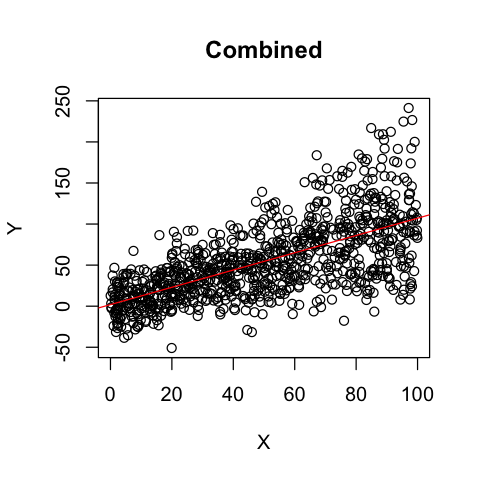In :
# Plotting separately
options(repr.plot.width=7, repr.plot.height=7)
par(mfrow=c(3, 3), mar=c(4, 4, 2, 1))

for (i in 1:9){
plot(mydata.df[Group==i, X1], mydata.df[Group==i, Y],
ylim=c(-100, 200), xlab='X', ylab='Y', main=paste('Group',i))
abline(lm(mydata.df[Group==i, Y] ~ mydata.df[Group==i, X1]), col='red')
}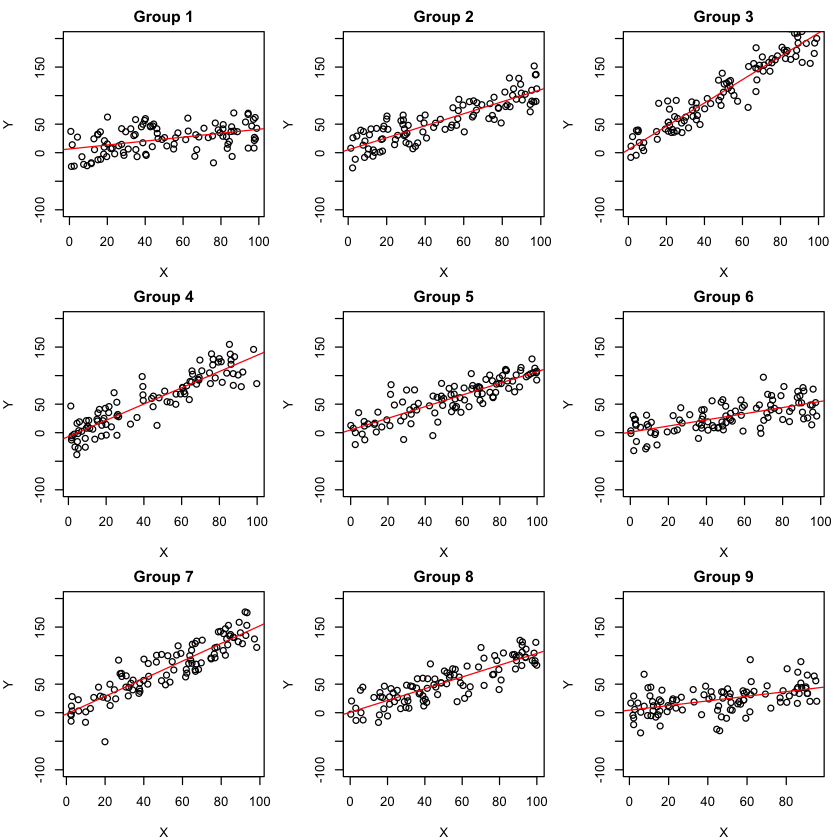Finally we need a way to build the augmented data matrix $\bs{X}_{(a)}$. To do that we will use the bdiag function from the Matrix package to implement sparse block diagonal matrices.

In :
# loading augmented matrix based on mydata
n <- dim(mydata) # column number
m <- unique(mydata[,n]) %>% length() # number of spatial regions
diags <- list() # saving device for diagonal matrices

for (i in 1:m){
diags[[i]] <- matrix(mydata[mydata[,n]==i,1:(n-2)], ncol=n-2)
}
return(bdiag(diags))
}

In :
# test
x0 <- rep(1, 6)
x1 <- rbinom(6, 10, 0.5)
y <- x0 + x1
group <- c(1,1,2,2,2,3)
XX <- cbind(x0, x1, y, group)
print(XX)

     x0 x1 y group
[1,]  1  6 7     1
[2,]  1  3 4     1
[3,]  1  4 5     2
[4,]  1  6 7     2
[5,]  1  4 5     2
[6,]  1  8 9     3

6 x 6 sparse Matrix of class "dgCMatrix"

[1,] 1 6 . . . .
[2,] 1 3 . . . .
[3,] . . 1 4 . .
[4,] . . 1 6 . .
[5,] . . 1 4 . .
[6,] . . . . 1 8

Now we are ready to initialize the model.

In :
X <- X.load(mydata) # load augmented sparse matrix
Y <- mydata[,3] # load response vector
print(dim(X)) # dimension of augmented matrix
print(length(Y)) # length of response vector

 900  18
 900

In :
# load permutation matrix P
print(P)

18 x 18 sparse Matrix of class "dgCMatrix"

[1,] 1 . . . . . . . . . . . . . . . . .
[2,] . . . . . . . . . 1 . . . . . . . .
[3,] . 1 . . . . . . . . . . . . . . . .
[4,] . . . . . . . . . . 1 . . . . . . .
[5,] . . 1 . . . . . . . . . . . . . . .
[6,] . . . . . . . . . . . 1 . . . . . .
[7,] . . . 1 . . . . . . . . . . . . . .
[8,] . . . . . . . . . . . . 1 . . . . .
[9,] . . . . 1 . . . . . . . . . . . . .
[10,] . . . . . . . . . . . . . 1 . . . .
[11,] . . . . . 1 . . . . . . . . . . . .
[12,] . . . . . . . . . . . . . . 1 . . .
[13,] . . . . . . 1 . . . . . . . . . . .
[14,] . . . . . . . . . . . . . . . 1 . .
[15,] . . . . . . . 1 . . . . . . . . . .
[16,] . . . . . . . . . . . . . . . . 1 .
[17,] . . . . . . . . 1 . . . . . . . . .
[18,] . . . . . . . . . . . . . . . . . 1


## Frequentist Estimation¶

The nice thing about augmented form is that we can attempt to fit a frequentist model using the glm function.

In :
# obtaining conbined data
df.aug <- as.data.table(cbind(as.matrix(X), Y))

V1V2V3V4V5V6V7V8V9V10V11V12V13V14V15V16V17V18Y
1 94.38393 0 0 0 0 0 0 0 0 0 0 0 0 0 0 0 0 69.171105
1 94.34750 0 0 0 0 0 0 0 0 0 0 0 0 0 0 0 0 67.039268
1 12.91590 0 0 0 0 0 0 0 0 0 0 0 0 0 0 0 0 24.337789
1 83.34488 0 0 0 0 0 0 0 0 0 0 0 0 0 0 0 0 7.560357
1 46.80185 0 0 0 0 0 0 0 0 0 0 0 0 0 0 0 0 24.744000
1 54.99837 0 0 0 0 0 0 0 0 0 0 0 0 0 0 0 0 6.479335
In :
model <- glm(Y ~ V1+V2+V4+V6+V8+V10+V12+V14+V16+V18, data = df.aug)
summary(model)

Call:
glm(formula = Y ~ V1 + V2 + V4 + V6 + V8 + V10 + V12 + V14 +
V16 + V18, data = df.aug)

Deviance Residuals:
Min       1Q   Median       3Q      Max
-82.010  -13.497   -0.317   13.749   63.534

Coefficients:
Estimate Std. Error t value Pr(>|t|)
(Intercept)  1.37712    1.37403   1.002    0.316
V1           5.17230    4.10185   1.261    0.208
V2           0.34564    0.06630   5.213 2.31e-07 ***
V4           1.10817    0.04002  27.690  < 2e-16 ***
V6           2.10205    0.03951  53.205  < 2e-16 ***
V8           1.29836    0.04313  30.102  < 2e-16 ***
V10          1.07191    0.03795  28.242  < 2e-16 ***
V12          0.53298    0.03934  13.548  < 2e-16 ***
V14          1.49107    0.03947  37.777  < 2e-16 ***
V16          1.02149    0.03951  25.853  < 2e-16 ***
V18          0.45361    0.04201  10.798  < 2e-16 ***
---
Signif. codes:  0 ‘***’ 0.001 ‘**’ 0.01 ‘*’ 0.05 ‘.’ 0.1 ‘ ’ 1

(Dispersion parameter for gaussian family taken to be 396.004)

Null deviance: 2030664  on 899  degrees of freedom
Residual deviance:  352048  on 889  degrees of freedom
AIC: 7950.3

Number of Fisher Scoring iterations: 2


Interesting! Clearly, the frequentist model is detecting the spatial random effect in the slope; however, it fails to detect the individual intercept terms. This is where Bayesian method prevails.

## Bayesian Estimation¶

In :
# initialize remaining variables
sig2 <- 0.2
tau2 <- 0.2
rho <- 2
abs <- c(10,10)
abt <- c(10,10)
alpha <- c(0.3, 0.3)
beta <- rep(1, 18)

In :
# Starting the model
beta.mcmc <- model.CAR(X, Y, rho, sig2, tau2, beta, P, D, W, abs, abt, alpha, N=10000, burnin=1000, thin=50)

 "SUMMARY"
 -------------------------
 "Iterations: 10000"
 "Burn-in: 1000"
 "Thin: 50"
 "Acceptance for sig2: 0.1608"
 "Acceptance for tau2: 0.1497"
 -------------------------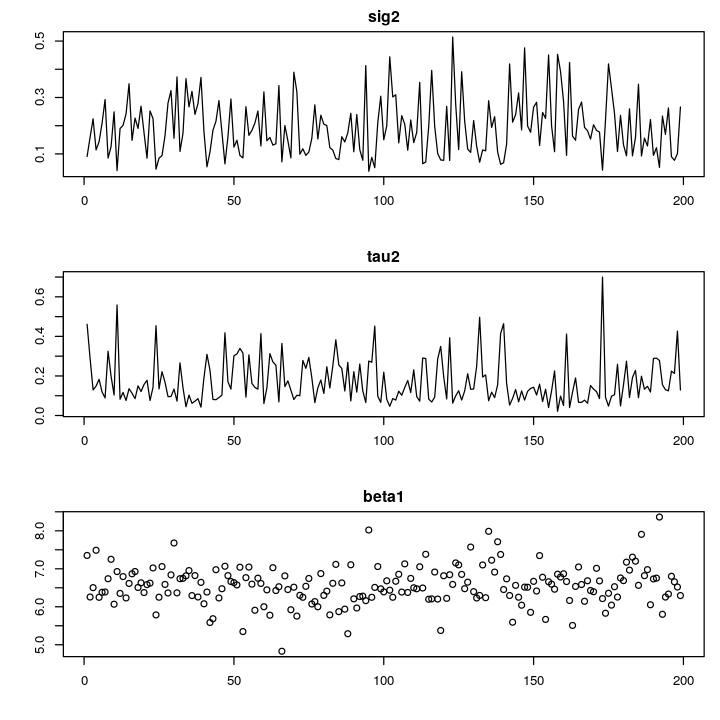In :
# Finding the posterior estimate of our parameters :D
beta.post <- colMeans(beta.mcmc)

In :
# Visualize first 4 components of beta
options(repr.plot.width=9, repr.plot.height=4)
par(mfrow=c(2, 2), mar=c(4, 4, 2, 1))
plot(beta.mcmc[,1], ylab="", xlab="", main=bquote(beta))
plot(beta.mcmc[,2], ylab="", xlab="", main=bquote(beta))
plot(beta.mcmc[,3], ylab="", xlab="", main=bquote(beta))
plot(beta.mcmc[,4], ylab="", xlab="", main=bquote(beta))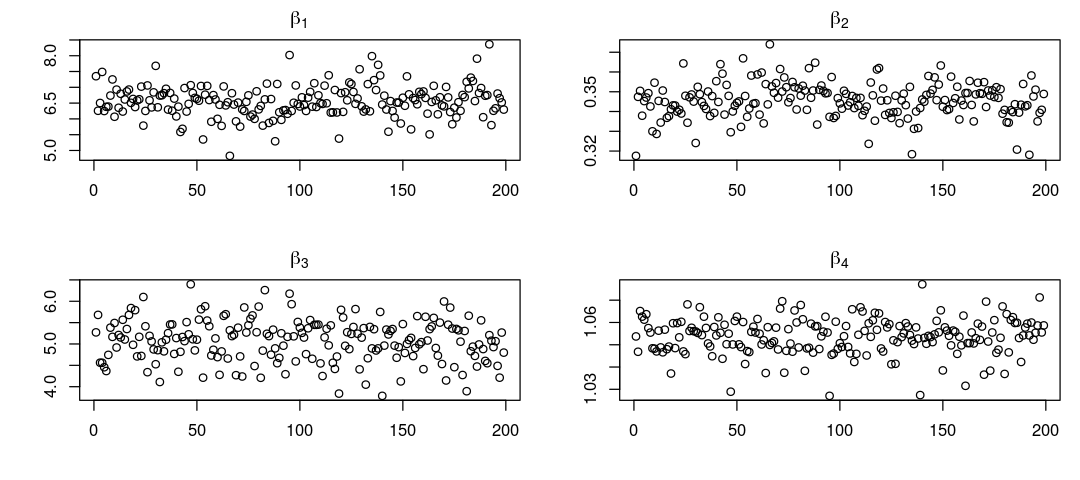Let's see how our model is doing by adding one more column as the predicted values.

In :
Y.pred <- X %*% beta.post %>% as.vector()
mydata.df <- cbind(mydata.df, Y.pred)

   X0       X1         Y Group   Y.pred
1:  1 94.38393 69.171105     1 39.16867
2:  1 94.34750 67.039268     1 39.15608
3:  1 12.91590 24.337789     1 11.01923
4:  1 83.34488  7.560357     1 35.35437
5:  1 46.80185 24.744000     1 22.72776
6:  1 54.99837  6.479335     1 25.55988

In :
# Plotting the prediction!
options(repr.plot.width=7, repr.plot.height=7)
par(mfrow=c(3, 3), mar=c(4, 4, 2, 1))

for (i in 1:9){
plot(mydata.df[Group==i, X1], mydata.df[Group==i, Y],
ylim=c(-100, 200), xlab='X', ylab='Y', main=paste('Group',i))
points(mydata.df[Group==i, X1], mydata.df[Group==i, Y.pred], ylim=c(-100, 200), col='red')
}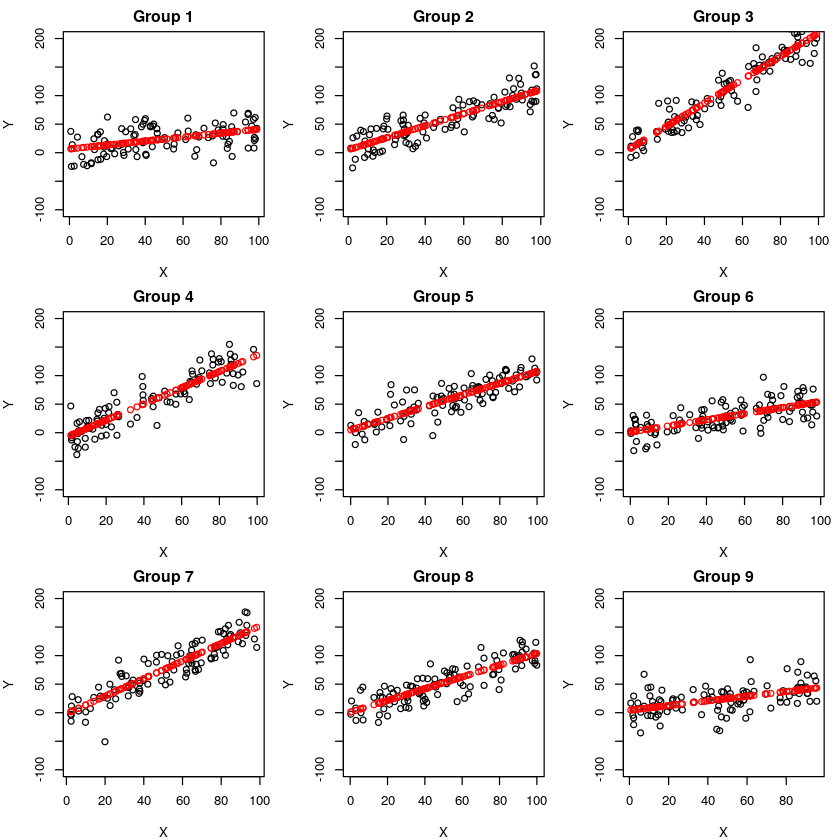We see that the slope parameters are very close to the frequentist estimation. In addition, the intercept is also corrected estimated.